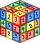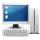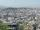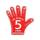# Five members

Write first 5 members geometric sequence and determine whether it is increasing or decreasing:
a1 = 3
q = -2

Result

a1 =  3
a2 =  -6
a3 =  12
a4 =  -24
a5 =  48

a5 =

#### Solution:Leave us a comment of example and its solution (i.e. if it is still somewhat unclear...):Be the first to comment!## Next similar examples:

1. Geometric sequence 4It is given geometric sequence a3 = 7 and a12 = 3. Calculate s23 (= sum of the first 23 members of the sequence).
2. GP - 8 itemsDetermine the first eight members of a geometric progression if a9=512, q=2
3. GP membersThe geometric sequence has 10 members. The last two members are 2 and -1. Which member is -1/16?
4. SequenceBetween numbers 1 and 53 insert n members of the arithmetic sequence that its sum is 702.
5. SequenceFind the common ratio of the sequence -3, -1.5, -0.75, -0.375, -0.1875. Ratio write as decimal number rounded to tenth.
6. Six termsFind the first six terms of the sequence a1 = -3, an = 2 * an-1
7. A perineumA perineum string is 10% shorter than its original string. The first string is 24, what is the 9th string or term?
8. Tenth memberCalculate the tenth member of geometric sequence when given: a1=1/2 and q=2
9. QuotientDetermine the quotient and the second member of the geometric progression where a3=10, a1+a2=-1,6 a1-a2=2,4.
10. Geometric progression 2There is geometric sequence with a1=5.7 and quotient q=-2.5. Calculate a17.
11. Geometric progression 48,4√2,4,2√2
12. ComputerThe computer was purchased 10000,-. Each year, the price of a computer depreciates always the same percentage of the previous year. After four years, the value of the computer is reduced to 1300,- How many percent was depreciated price of the computer each
13. The cityAt the end of 2010 the city had 248000 residents. The population increased by 2.5% each year. What is the population at the end of 2013?
14. DiscriminantDetermine the discriminant of the equation: ?
15. Theorem proveWe want to prove the sentence: If the natural number n is divisible by six, then n is divisible by three. From what assumption we started?
16. ValueFind the value of the expression: 6!·10^-3
17. One halfOne half of ? is: ?